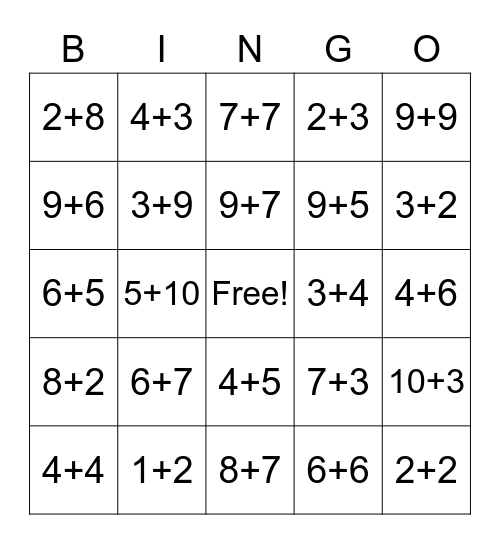This bingo card has a free space and 44 words: 1+1, 2+2, 3+3, 4+4, 5+5, 6+6, 7+7, 8+8, 9+9, 1+2, 2+3, 3+4, 4+5, 6+7, 7+8, 8+7, 7+6, 6+5, 5+4, 3+2, 4+3, 2+1, 1+9, 2+8, 3+7, 4+6, 5+5, 6+4, 7+3, 8+2, 9+1, 10+3, 3+10, 4+10, 5+10, 7+10, 8+10, 9+10, 9+7, 9+6, 9+5, 4+9, 3+9 and 2+9.

⚠ This card has duplicate items: 5+5 (2)

## Play Online